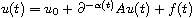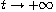Electron. J. Differential Equations, Vol. 2018 (2018), No. 173, pp. 1-27.

### Well-posedness, regularity, and asymptotic behavior of continuous and discrete solutions of linear fractional integro-differential equations with time-dependent order Eduardo Cuesta, Rodrigo Ponce

Abstract:
We study the well-posedness of abstract time evolution fractional integro-differential equations of variable order. Also we study the asymptotic behavior as, and the regularity of solutions. Moreover, we present the asymptotic behavior of the discrete solution provided by a numerical method based on convolution quadratures, inherited from the behavior of the continuous solution. In this equation A plays the role of a linear operator of sectorial type. Several definitions proposed in the literature for the fractional integral of variable order are discussed, and the differences between the solutions provided for each of them are illustrated numerically. The definition we chose for this work is based on the Laplace transform, and we discuss the reasons for this choice.

Submitted April 27, 2018. Published October 17, 2018.
Math Subject Classifications: 45A05, 45E10, 45N05, 65R20, 65J08, 65J10.
Key Words: Fractional integrals; Banach spaces; variable order; convolution quadratures.

Show me the PDF file (597 KB), TEX file for this article.Eduardo Cuesta Department of Applied Mathematics E.T.S.I. of Telecomunication Campus Miguel Delibes University of Valladolid 47011, Spain email: eduardo@mat.uva.es Rodrigo Ponce Universidad de Talca Instituto de Matemática y Física Casilla 747, Talca, Chile email: rponce@inst-mat.utalca.cl